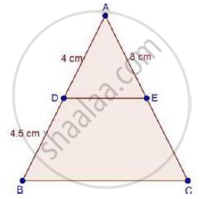Share

# In δAbc, D and E Are Points on the Sides Ab and Ac Respectively Such that De || Bc If Ad = 4 Cm, Db = 4.5 Cm and Ae = 8 Cm, Find Ac. - Mathematics

Course

#### Question

In ΔABC, D and E are points on the sides AB and AC respectively such that DE || BC

If AD = 4 cm, DB = 4.5 cm and AE = 8 cm, find AC.

#### SolutionWe have,

DE || BC

Therefore, by basic proportionality theorem, we have,

"AD"/"DB"="AE"/"EC"

rArr4/4.5=8/"EC"

rArr"EC"=(8xx4.5)/4

⇒ EC = 9cm

Now, AC = AE + EC

= 8 + 9

= 17 cm

∴ AC = 17 cm

Is there an error in this question or solution?

#### APPEARS IN

RD Sharma Solution for Class 10 Maths (2018 (Latest))
Chapter 7: Triangles
Ex. 7.2 | Q: 1.06 | Page no. 19
RD Sharma Solution for Class 10 Maths (2018 (Latest))
Chapter 7: Triangles
Ex. 7.2 | Q: 1.06 | Page no. 19

#### Video TutorialsVIEW ALL 

Solution In δAbc, D and E Are Points on the Sides Ab and Ac Respectively Such that De || Bc If Ad = 4 Cm, Db = 4.5 Cm and Ae = 8 Cm, Find Ac. Concept: Basic Proportionality Theorem Or Thales Theorem.
S